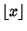## Digit

The number of digitsin an Integeris the number of numbers in some base (usually 10) required to represent it. The numbers 1 to 9 are therefore single digits, while the numbers 10 to 99 are double digits. Terms such as double-digit inflation'' are occasionally encountered, although this particular usage has thankfully not been needed in the U.S. for some time. The number of (base 10) digits in a numbercan be calculated aswhereis the Floor Function.